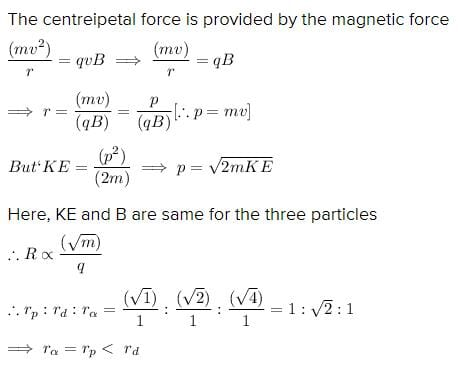JEE  >   QUIZ 2:Magnetism (#Free Test Series)

# QUIZ 2:Magnetism (#Free Test Series)

Test Description

## 5 Questions MCQ Test | QUIZ 2:Magnetism (#Free Test Series)

QUIZ 2:Magnetism (#Free Test Series) for JEE 2022 is part of JEE preparation. The QUIZ 2:Magnetism (#Free Test Series) questions and answers have been prepared according to the JEE exam syllabus.The QUIZ 2:Magnetism (#Free Test Series) MCQs are made for JEE 2022 Exam. Find important definitions, questions, notes, meanings, examples, exercises, MCQs and online tests for QUIZ 2:Magnetism (#Free Test Series) below.
Solutions of QUIZ 2:Magnetism (#Free Test Series) questions in English are available as part of our course for JEE & QUIZ 2:Magnetism (#Free Test Series) solutions in Hindi for JEE course. Download more important topics, notes, lectures and mock test series for JEE Exam by signing up for free. Attempt QUIZ 2:Magnetism (#Free Test Series) | 5 questions in 15 minutes | Mock test for JEE preparation | Free important questions MCQ to study for JEE Exam | Download free PDF with solutions
 1 Crore+ students have signed up on EduRev. Have you?
QUIZ 2:Magnetism (#Free Test Series) - Question 1

### Two particles, each of mass m and charge q , are attached to the ends of a light rigid rod of length 2R. The rod is rotated at constant angular speed about a perpendicular axis passing through its center. The ratio of the magnitudes of the magnetic moment of the system and its angular momentum about the center of the rod is

QUIZ 2:Magnetism (#Free Test Series) - Question 2

### a current I flows along the length of an infinitely long, straight, thin-walled pipe. Then

QUIZ 2:Magnetism (#Free Test Series) - Question 3

### A battery is connected between two points A and B on the circumference of a uniform conducting ring of radius r and resistance R. One of the arcs AB of the ring subtends an angle θ at the center . The value of the magnetic induction at the center due to the current in the ring is

QUIZ 2:Magnetism (#Free Test Series) - Question 4

Two particles X and Y having equal charges, after being accelerated through the same potential difference, enter a region of uniform magnetic field and describe circular paths of radii R1 and R2 respectively.  The ratio of the mass of X to that of Y is

QUIZ 2:Magnetism (#Free Test Series) - Question 5

A proton, a deutron and an α-particle having the same kinetic energy are moving in a circular trajectories in a constant magnetic field. If rp , rd and rα denote, respectively the radii of the trajectories of these particles, then

Detailed Solution for QUIZ 2:Magnetism (#Free Test Series) - Question 5Use Code STAYHOME200 and get INR 200 additional OFF Use Coupon Code
Information about QUIZ 2:Magnetism (#Free Test Series) Page
In this test you can find the Exam questions for QUIZ 2:Magnetism (#Free Test Series) solved & explained in the simplest way possible. Besides giving Questions and answers for QUIZ 2:Magnetism (#Free Test Series), EduRev gives you an ample number of Online tests for practice